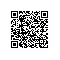# 《Java程序设计习题精析与实验指导》一2.2　习题解析

### 2.2　习题解析

1. 在Java程序设计语言中，下面______类型可以表示整数基本数据类型。
A. single B. byte C. double D. char

1. float型数据在计算机内所占用的存储空间为______字节。
A. 2 B. 4 C. 8 D. 16

1. 下面______是Java数据类型中int型的取值范围。
A. –27～27–1 B. 0～216–1 C. –215～215–1 D. –231～231–1

1. 以下字符常量中不合法的是______。
A. '#' B. '&' C."P" D. '数'

1. 以下声明的变量中，______是不合法的。
A. inter_net B. i_nong C. Hello D. *member

1. 以下选项中的变量都已正确定义并且已被赋初值，不合法的表达式是______。
A. m >= 6 = = n>1 B. 'm'+6 C. 'm'=8 D. 'M'%8

1. 设m、n、x、y为已赋值的 int 型变量，下列______的运算结果属于非逻辑值。
A. m!=n & x%y < m B. m++ = =m+n+x+y

C. ++m*b––+y D. m+n>=x+y

1. 定义a为int类型的变量并且已被赋初值，则合法的赋值语句是______。
A. a +1==4; B. a+=a*8; C. a=6.6f; D. int a=18;

1. 有下列语句：
2. e=Integer. parseInt(""+3+3);

System.out.println(e-2.5);

A. 30.5 B. 3.5 C. 332.5 D. 8.5

1. 执行下列程序段：
2. no;

int a=19,b=8;
no=(a%b>5)?a+b:a-b;
System.out.println(no);

A. 2 B. 9 C. 11 D. 27

1. 下面的程序段执行后的结论是______。
2. m=2,n=2

m+=m-=m*m;
n-=n*n;
n=n+n;
A. m=n B. m>n C. m答案：B

1. 下列______方法不属于java.lang.Math类（方法名相同即可）。
A. sin() B. sqr() C. abs() D. random()public class Dogs {
public static void main(String[] args) {
int n=2;
System.out.println("我有 "+    (1)    +"只狗");
System.out.println("你有 "+    (2)    +"只狗");
}
} 

（2）(n+1)